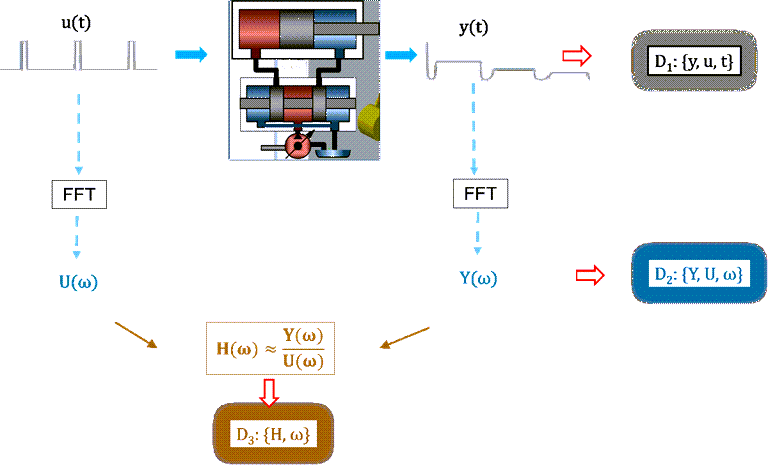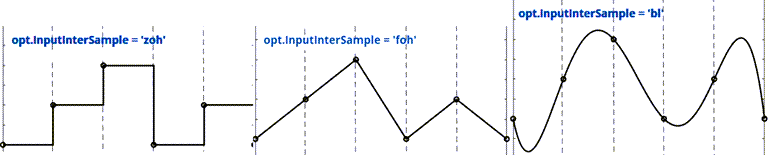## Data Domains and Data Types in System Identification Toolbox

System Identification Toolbox™ uses measured input and output data to estimate the parameters of a variety of dynamic models. This estimation data can be in the form of time-domain data, frequency-domain data, or frequency response data.

• Time-Domain Data — Input and output signal values corresponding to a time vector. You can identify both linear and nonlinear models using time-domain data. You identify time series models using only the output signals of time-domain data.

• Frequency-Domain Data — FFT of time-domain signals. Frequency-domain data is typically used for linear model identification, as using this type of data allows data compression and faster estimation algorithms than in the time domain.

• Frequency Response Data (FRD) — Gain and phase of the system output relative to its input, recorded over a range of sinusoidal inputs of varying frequencies. Frequency response data is also known as the frequency response function, or FRF. Like frequency-domain data, the FRD type provides the benefit of data compression and fast estimation algorithms, and is used for identifying linear models.

For most estimation functions, the data must be uniformly sampled in time.

### Generating Data in Different Time and Frequency Domains

The following schematic diagram illustrates the data generation process for the three domain types.1. Signals originate in the time domain where the system or process to be modeled is excited by an external stimulus—the input u(t)—and the corresponding response of the system—the output y(t)—is recorded. These signals are recorded over a finite span of time t and result in vectors or matrices that store the measured values. The triplet of recorded values {y,u,t}, shown as D1 in the schematic diagram, forms the time-domain data.

An additional piece of information from the stimulus/response measurement process is the intersample behavior between the discrete sample points, which the stimulus generation method for that process defines. For instance, at each sample point, the stimulus generator may instantaneously raise the input value and then hold that value constant until the next sample point. This approach is called zero-order hold. The schematic diagram depicts this behavior in the pulse sequence for u(t). If the generator instead ramps the input value from one sample point to the next, this approach is called first-order hold, as illustrated, along with the other intersample options, in the following figure. Knowing the intersample behavior is important when you are identifying continuous systems and need to know what happens in between the discrete measurements.2. The time-domain signals can be converted into their frequency-domain counterparts by using a discrete Fourier transform, typically with an FFT operation. This operation requires the time-domain signals to be collected on a uniform time grid, that is, made available at a constant sampling interval Ts. Unless zero-padding is used, the resulting signals correspond to a uniform frequency grid of as many samples as in the original time-domain signals, spaced over a range of 0 to the Nyquist frequency π/Ts, where Ts is the time-domain data sampling period. The triplet {Y,U,ω} is called frequency-domain data and is shown as D2 in the schematic diagram. To make the frequency-domain data independent of any correspondence to another time-domain data set, the sample time Ts is also stored.

3. The dynamic behavior of a system is often described by its frequency response, which is a set of the gain and phase values of the output of the system relative to a sinusoidal input over a range of input frequencies. Typically, hardware spectrum analyzers compute these values. The values can also be computed by applying spectral analysis techniques to the time-domain or frequency-domain data. The result is a complex frequency response vector H(ω) computed over a range of ω values. The pair {H,ω} is called the frequency response data (FRD) or frequency response function (FRF), shown as D3 in the schematic diagram.

Most estimation functions support estimation data sets that contain an arbitrary number of input and output signals. Exceptions are functions that are specific to a more restrictive model, such as a time series model that uses only output signals. In these cases, rather than modify the estimation data set itself, you can select specific channels to use when you configure the estimation command. For example, you might have a data set that contains both input and output data but you want to generate a time series model from that data. When you perform the estimation, you can specify that only the output channels of the original input/output data set be used.

### Data Types

As of R2022b, System Identification Toolbox supports three data types for representing time-domain data: timetables, numeric matrices, and `iddata` objects.

#### Timetables

Timetables are a built-in MATLAB® data type represented by the `timetable` object. Timetables are used to represent observations as variables that are ordered by time. For system identification, these variables represent the input and output channel data, and can be used for time-domain identification. You can create timetables by using the timetable constructor and convert matrices to timetables by using the `array2timetable` command. To convert an `iddata` to a timetable, use `iddata2timetable`. In addition to the observation data, timetables store a limited number of attributes, or properties, that provide descriptive information about the data.

#### Numeric Matrices

Numeric matrices represent observations ordered by time but do not contain explicit time or sample time information. The observations are along the rows and the channels are along the columns. Numeric matrices provide the simplest data format and are often sufficient for identifying discrete-time models. However, because this format does not supply time information, the use of numeric matrices for continuous-time model estimation is not recommended.

The most common syntax for estimating with numeric matrices is to use separate arguments for the input and the output matrices. For more information about using matrix-based data, see Use Matrix-Based Data for Time-Domain System Identification.

#### `iddata` Objects

The `iddata` object is unique to System identification Toolbox and provides a comprehensive set of information about the estimation data. In an `iddata` object, you can store the measured signals, their sampling information, names and units, experiment descriptions, and other attributes in a single object. The `iddata` object can be used to represent both time-domain and frequency-domain data.

Historically, the `iddata` object was the primary data format for system identification. However, because the use of `iddata` objects is limited to system identification, these objects cannot be used in other toolboxes and are not as familiar to users who have experience in other toolboxes. For these reasons, as of R2022b, the timetable is now the primary format for time-domain estimation, since timetables can be used in multiple toolboxes. If you want to represent frequency-domain data, you still must use the `iddata` object.

One limitation of the `iddata` object is that all the packaged signals must share a common time vector. All estimation functions support this format, and most of them require it. However, as new functionalities evolve which require more flexible data support, such as neural state-space model estimation, this `iddata` object limitation imposes a limitation on estimation possibilities.

For more information about `iddata` objects, see Representing Time- and Frequency-Domain Data Using iddata Objects.

#### `idfrd` Objects

The `idfrd` object, like the `iddata` object, is unique to System Identification. However, the object is similar to the `frd` (Control System Toolbox) object. The `idfrd` object is used to store measured frequency response data. The location of the required data content of the object depends on whether the model being estimated is an input/output model or a time series model:

• Input/output model — `ResponseData` and `Frequency` properties

• Time series model — `SpectrumData` and `Frequency` properties

If the frequency response data comes directly from hardware, such as by using a spectrum analyzer, set the data sample time to zero (```G = idfrd(response, frequency, 0)```). If the frequency response was obtained by converting a time-domain data set, the sample time of the `idfrd` object matches that of the original data. You can set the `idfrd` sample time to zero, which results in a continuous-time model, only if the original data used band-limited inputs. Note that setting the `idfrd` sample time to zero is desirable since it allows you to identify continuous-time parametric models with high speed and accuracy.

For more information about `idfrd` objects, see Representing Frequency-Response Data Using idfrd Objects.